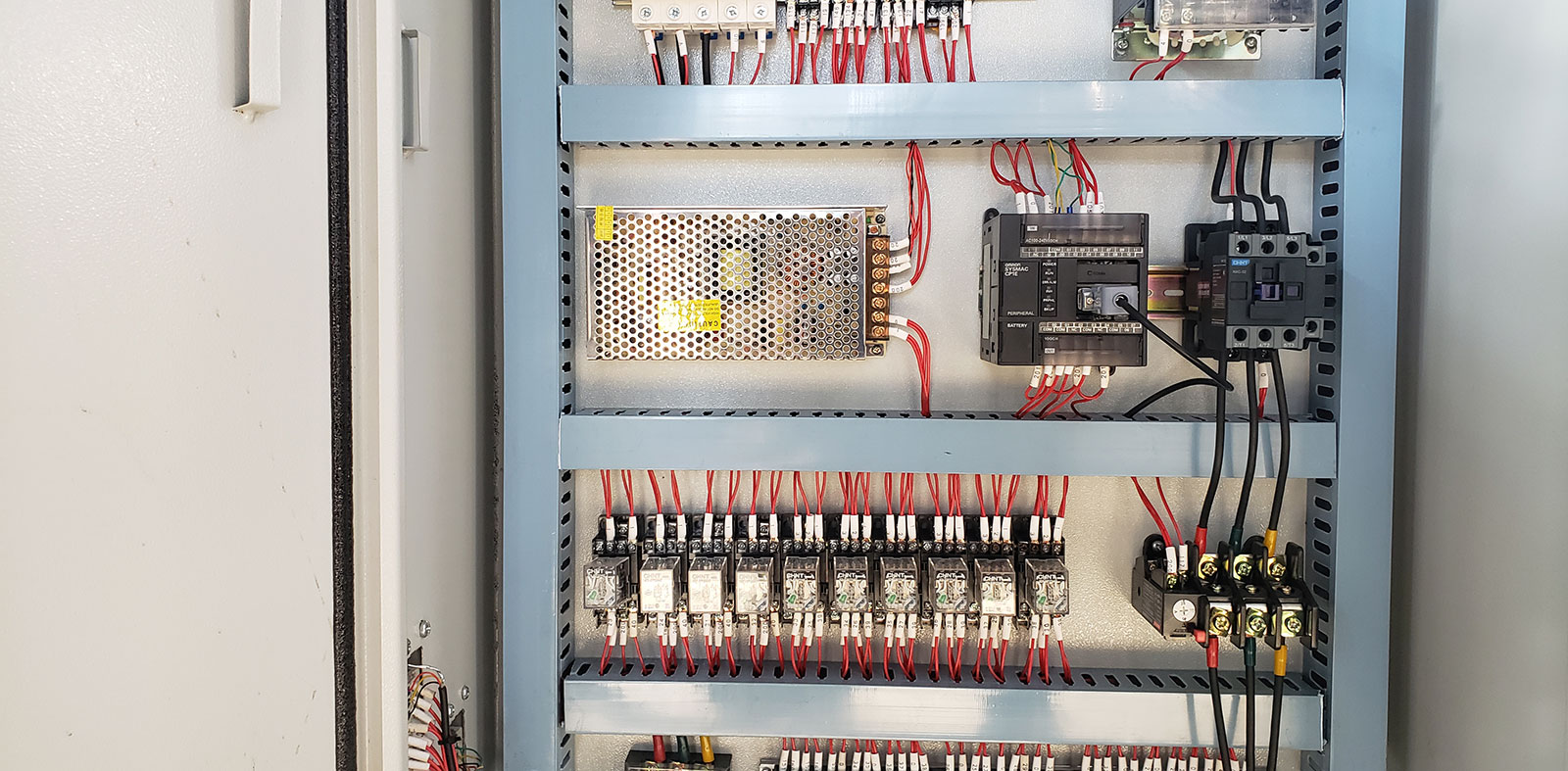> > Electrical circuit diagram of profile bending machine

# Electrical circuit diagram of profile bending machine### Introduction

The electrical control principle diagram adopts the circuit numbering method, which means that each contact in the circuit is numbered with letters or numbers.

#### Note the following two points when numbering profile bending machines.

1. the main circuit three sets the AC power lead-in line identification mark, and the neutral line uses the N mark to mark the mark. At the outlet of the power switch. Then press from top to bottom, from left to right. After each electrical component is turned, the number will increase, such as U12, V12, W12, U13, V13, W13, and a single three-order AC motor (or equipment The three lead wires of) are numbered u, V, w in sequence according to the phase sequence. For the number of lead wires of multiple motors, in order not to cause mixing, they can be distinguished by different numbers before the letters, such as 1U, 1V, 1W, 2U, 2V, 2W

2. The auxiliary circuit number is numbered sequentially from top to bottom and from left to right according to the “equipotential” criterion. After each electrical component is turned, the number shall be sequentially increased. The starting number of the control circuit number must be 1, and the starting numbers of other auxiliary circuit numbers are incremented by 100. For example, the lighting circuit number starts from 101, and the indicating circuit number starts from 201.

1. Marking of the technical data in the circuit diagram of the profile bending machine. Generally, the following content is also marked in the circuit diagram. The voltage value, polarity or frequency and phase number of each power circuit; the characteristics of certain components, such as the value of resistance and capacitance; and the operation methods and functions of uncommon electrical appliances.

Further reading:The key points must be known before operation of the profile bending machine